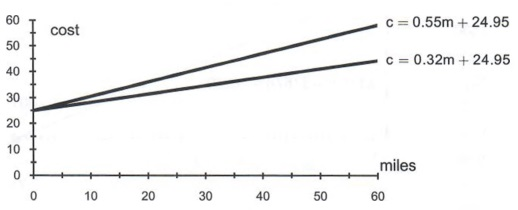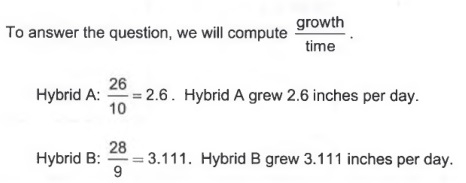Algebra

Equations

Inequalities

Graphs

Numbers

Calculus

Matrices

Tutorials

Enter the expression you want to simplify, set the options and click the Simplify button.

Expression
Options
 Trig functions
 Simplify fully
Output Style
 Graphic image
 Text

# Basic arithmetic and algebraic simplification

## SIGNED NUMBERS

### Objectives

In this section, you will add, subtract, multiply and divide signed numbers. Also in solving arithmetic expression, you will use the order of operations including exponents.

### Intuitive Approach to Addition and Subtraction:

Example 1. I have \$40, and I owe you \$75. What is my net worth?

Having \$40 is equivalent to + 40.
Owing \$75 is equivalent to - 75.
My net worth will be indicated by 40 - 75.
or
40 - 75 = -35
My net worth is -\$35.

Since I owe more than I have, the answer has to be a negative number.

Rule: Intuitive Rule for combining numbers with unlike signs:
Find the difference (subtraction) of the two numbers and use the sign of the larger number.

A way to use this rule is to cover the signs of the numbers. Find the difference between the numbers with the signs covered. Use the sign of the larger number.

Example 2. I am in debt for \$50, and I owe you \$60. What is my net worth?

Being in debt for \$50 is equivalent to -50.
Owing \$60 is equivalent to - 60.
My net worth is indicated by -50 - 60.
or
-50-60 = -110
My net worth is -\$110.

Since I am in debt and I owe you money, my net worth has to be Negative.

Rule: Intuitive Rule for combining numbers with like signs: Add the two numbers and use the common sign.

Study Tip: Make a note card with the rules for adding and subtracting like and unlike signed numbers. Review note cards at least twice a week as part of your homework routine.

### Multiplication and Division:

Vocabulary: The product is the answer to a multiplication problem.
The quotient is the answer to a division problem.

Example 3. I will lose \$9 a day for each of the next 6 days. How much money will I lose?

Losing \$9 is equivalent to -9.
Since I am losing \$9 everyday for the next 6 days, I will have -9 * 6 fewer dollars.
or
-9 * 6 = -54
I will lose \$54 in the next 6 days.

Since I am losing money, the answer has to be a negative number.

In the example above, -54 is the product.

Vocabulary: The factors are the numbers being multiplied. In the example above, -9 * 6 = -54 , -9 and 6 are the factors.

Rule: The product or quotient of two numbers with unlike signs is always negative.

Example 4. I lost \$8 a day for the previous 7 days. How much more money did I have 7 days ago?

Since I had more money 7 days ago, the answer has to be a positive number.

To find the answer: Losing \$8 is equivalent to -8. Previous 7 days is equivalent to -7 Since I have lost \$8 everyday for the past 7 days, I will have -8 * -7 fewer dollars. or -8 * -7 = 56 I had 56 more dollars 7 days ago.

Rule: The product or quotient of two numbers with like signs is always positive.

Example 5. Divide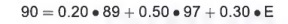Since the numerator and denominator are both negative, the quotient must be positive.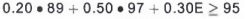Study Tip: Make note cards for all of the rules and vocabulary in the course.

Multiplication problems can be expressed in several ways: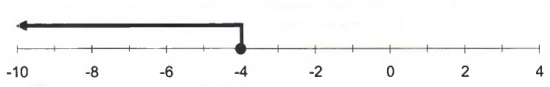Division problems can be expressed in several ways: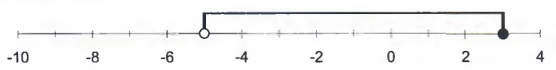### Zero in a Division Problem

Division can be checked using multiplication. For example,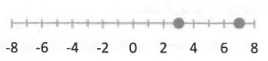because 18 = 3 * 6

18 divided by 6 is the same as asking what number multiplied by 6 equals 18?

How does zero in the numerator affect a division problem?

For example,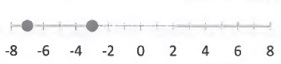is the same as asking, what number multiplied by 31 equals 0? So,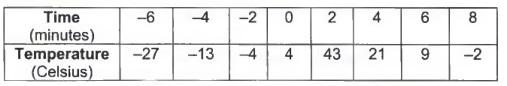= 0 because 0 * 31 = 0.

Zero divided by any number equals zero.

How does zero in the denominator affect a division problem?

For example,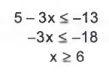is the same as asking, what number multiplied by 0 equals 17? A number doesn't exist that when multiplied by 0 is 17. Thus, the answer tois undefined.

Any number divided by zero is undefined.

How does zero in both the numerator and denominator affect a division problem?

For example,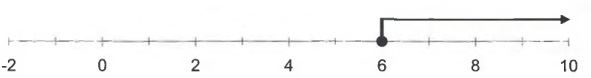is the same as asking, what number multiplied by 0 equals 0? Five multiplied by zero equals zero, so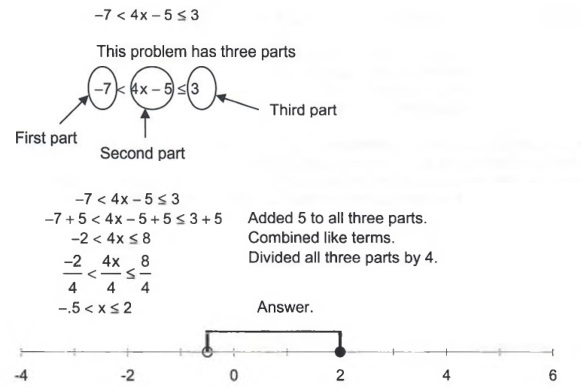. Also, 3 multiplied by zero equals zero, so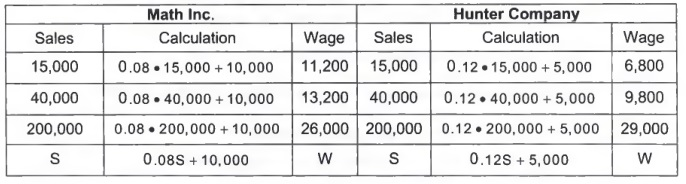.

In fact, every number multiplied by zero equals zero, soequals any every number.

Zero divided by zero can not be uniquely determined and is called indeterminate.

Study Tip: Division by zero (zero in the denominator) is undefined. Zero in both numerator and denominator is called indeterminate. Put these concepts on a note card.

Vocabulary: 3 and -3 are opposites because they both are the same distance from zero on the number line but in opposite directions. They are also called additive inverses because their sum is zero.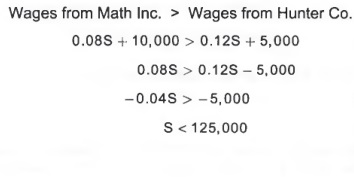The opposite of -6 is 6. In mathematical notation, - (-6) = 6. The first negative sign means to use the opposite; the second negative sign means that 6 is negative or to the left of zero on the number line.

In the application below, the word "profit" is used even if the number is negative, which really indicates a loss.

Example 6. An Application: The graph below shows the profit and loss of SRH Inc. for the years 2001 through 2006.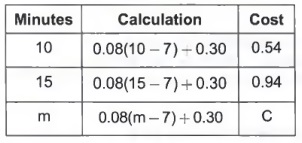a. What was the difference between the profit in 2005 and the loss in 2002?

"Difference" means subtraction. So to answer the question, subtract the loss in 2002 from the profit in 2005:

(2005 profit) - (2002 loss).
7.3 - (-15.8)
From the chart, find the appropriate profit and loss.
= 7.3 + 15.8
Interpret - (-15.8) as finding the opposite of -15.8.
= 23.1

The difference between the profit in 2005 and the loss in 2002 was 23.1 million dollars. The answer is positive because SRH's profits increased from year 2002 to 2005.

b.. What was the difference between the profit in 2006 and profit in 2005? What is the significance of the negative sign in your answer?

(profit in 2006) - (profit in 2005)
5.2-7.3= -2.1
The difference in profit was -2.1 million dollars.

The answer is negative because SRH's profits decreased from year 2005 to 2006.

c. What is the mean (average) profit for the six years?

To find the average, add the profits and losses; then divide by the number of years.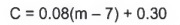The average profit for the six years was a loss of 5.317 million dollars or -5.317 million dollars in profit.

Using a calculator to add, subtract, multiply, or divide:

(The TI-30X II S calculator is recommended for the course. See the MAT 011 Electronic Resource page to learn how to use the calculator.)

The keys for multiplication, addition, and division are the standard ones. Note that the key for multiplication on the calculator is x, but it appears as * on the calculator screen. For division, the division key is +, but appears as / on the screen.

Example 7. - 7.4 - 8.6 = -16
- 7.4 indicates that 7.4 is negative. To input a negative number into a calculator, you must use the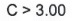key which is different from the subtraction key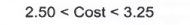Study Tip: Before using a calculator, you should take a couple of seconds to mentally estimate the answer.

Vocabulary: Exponents: bn means that the number b is used as a factor n times.

Example 8. (-7)2 = (-7)(-7) = 49

### Order of Operations:

When a numerical algebra problem has more than one operation, the order is as follows:

First: Inside Parentheses, ().
Second: Exponents
Third: Multiplication or Division (left to right)
Fourth: Addition or Subtraction (left to right)

Study Tip: Please Excuse My Dear Aunt Sally is a mnemonic used to learn the order of operations. P (Parentheses), E (Exponents), M (Multiplication), D (Division), A (Addition), and S (Subtraction).

Example 9. -6(8 - 13) = -6(-5) = 30
Add the signed numbers inside the parentheses. The product of numbers with like signs is positive.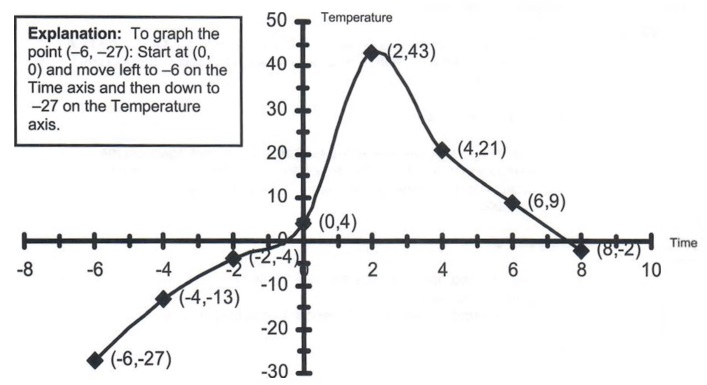Compute the quantity (-3)2.
Compute the opposite of +9.
Subtract using the rules of signed numbers.

Estimation: It is important to estimate the result before using a calculator. This will help you determine if your answer is reasonable. Estimation should be quick and performed mentally. The key to estimating is rounding.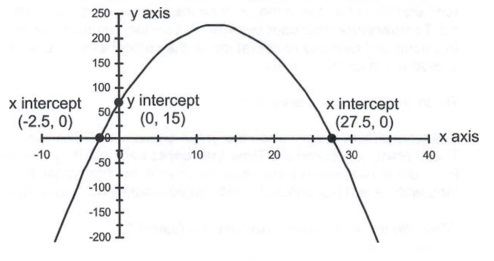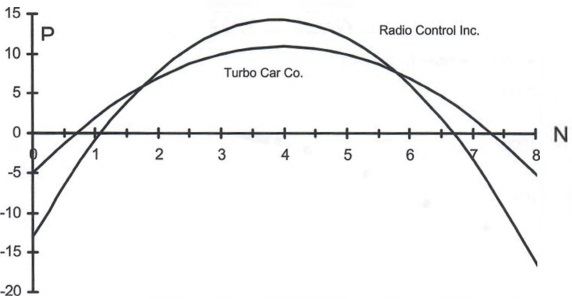### Summary:

Signed numbers are a key concept in Beginning Algebra. Initially, they can be confusing, but once the rules are learned and practiced, these numbers function in very predictable ways. Along with signed numbers, the order of operations must be mastered early in the semester. Answers will vary dramatically if the correct order of operations is not followed.

1. Intuitive rules for adding and subtracting signed numbers:
a. Unlike Signs: Find the difference (subtraction) of the two numbers and use the sign of the larger number.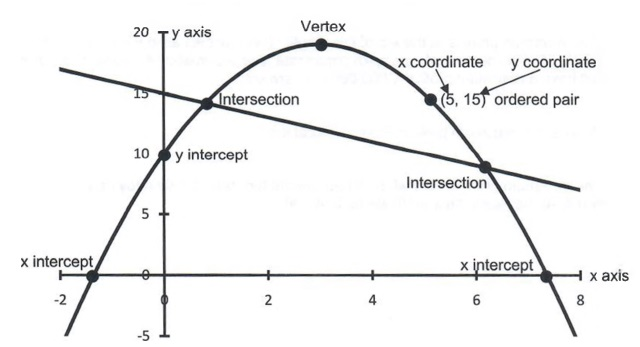b. Like Signs: Add the two numbers and use the common sign.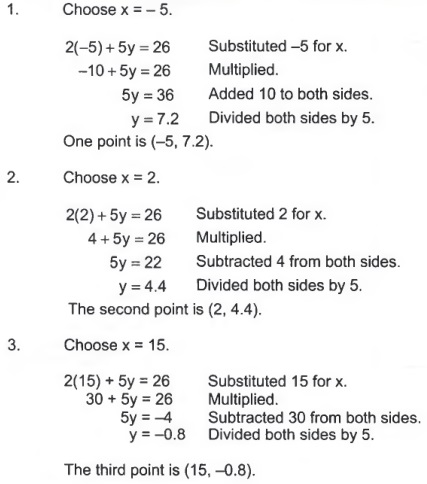2. Rules for multiplying and dividing two signed numbers:
a. Unlike signs: The result is always negative.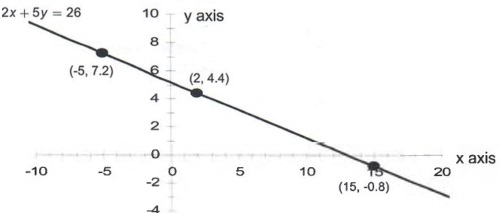b. Like signs: The result is always positive.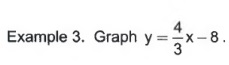3. Order of Operations:
First: Inside parentheses, ()
Second: Exponents
Third: Multiplication and Division (left to right)
Fourth: Addition and Subtraction (left to right)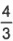## INTRODUCTION TO VARIABLES

### Objectives:

By performing similar arithmetic steps, you will discover the need for variables.

Example 1. You need to rent a moving van. Class Movers charges a basic rate of \$24.95 plus 32 cents per mile.

a. Calculate the cost of renting a van if you drive the following miles: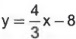Vocabulary: A variable in algebra is a letter that represents a quantity that can change. In the example above, m represents the number of miles, and c represents the cost. Both miles and cost can vary or change. A variable term contains a letter and a number multiplying it; 0.32m is a variable term. A constant is a number that never changes value; 24.95 is a constant.

b. What is the equation that relates cost and number of miles driven? The last row in the table above contains the answer, c = 0.32m + 24.95

c. Another rental company, Zippo Movers, charges a flat rate of \$42.95. How many miles would you have to drive for Zippo and Class to charge the same?

The cost equation for Zippo is: c = 42.95

To calculate when the two companies' charges are the same, set their cost equations equal to each other.

Cost of Zippo = Cost of Class
42.95 = 0.32m+ 24.95

Because we have only just begun to study algebra, we will guess at the solution. Substitute a guess for the number of miles into the equation for Class Movers,

c = 0.32m+ 24.95.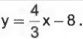If you drive 55 miles, then the two companies will charge about the same. Guessing is very tedious and not precise. Later in the chapter, we will use algebra to solve the problem. Algebra is direct and is more precise than guessing.

Example 2. It is estimated that a 2011 Toyota Sienna Minivan loses \$1,800 a year in value. The minivan originally cost \$42,000.

a. Calculate the value of the minivan for the following years.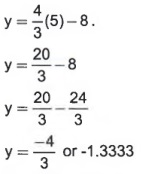b. What is the equation that relates the value of the van and the number of years since 2011?

The last row in the table above contains the answer, v = 42,000 - 1,800t

c. When will the van be worth \$20,400?

To answer the question, you must find a value for y that will make v = 20,400 or

20,400 = 42,000 - 1800t.

Since we don't know how to solve the problem using algebra yet, we will guess at the solution. Substitute a guess for t, the number of years since 2007, into the equation

V = 42,000 - 1,800t.

Repeatedly doing this will generate the following table.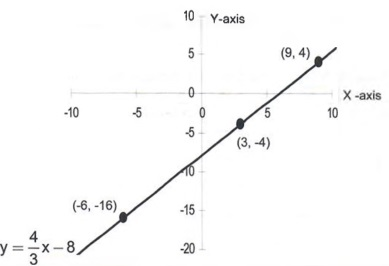The minivan will be worth \$20,400 twelve years after 2011. In year 2023, the minivan will be worth \$20,400

### Summary:

Learning to generate a table is another key step in beginning algebra. Be sure your table contains the correct number of columns for the information you need. Use a ruler to draw the tables to ensure the information does not get confusing.

For every problem in this section you should be able to:

1. Generate a table.

Explanation: The calculation column is the most important. It indicates how you get your equation.

2. When you have determined the equation, you should understand what each term of the equation represents.

For example: c = 0.32m + 24.95 * c is the cost of renting a moving van. * 0.32 is the amount Class Movers charges per mile, m is the number of miles you drive the van. * * 24.95 is the basic rate or fixed cost.

Study Tip: Use descriptive letters for variables in application problems, used c for cost and m for number of miles driven.

## SIMPLIFLYING ALGEBRAIC EXPRESSIONS

### Objectives

This section begins the process of solving equations. You will understand how to combine like terms and how to use the distributive property.

Vocabulary: Terms: parts of an algebraic expression separated by addition or subtraction signs.

Example 1. For 3 + 5 = 8, 3 and 5 are terms.
Example 2. For 3x - 5y, 3x and -5y are terms.

Coefficient: the number multiplying the variable.

Example 3. For the expression 7x - 2y + z,
7 is the coefficient of x;
-2 is the coefficient of y, and
1 is understood to be the coefficient of z.

Like Terms: terms which have the same variable and exponent; and terms which are numbers without variables.

Example 4. For the expression 4x + 7y - 3x + 4z, 4x and -3x are like terms.

Rule: To combine like terms, add their coefficients. 4x + (-3x) = x or 1x.

Example 5. Combine like terms.
3x - 5 - 6x + 7 = -3x + 2
Identify the like terms, 3x and -6x, -5 and 7.
Add the coefficients of the like terms.

Vocabulary: Distributive Property: Definition a(b + c) = a * b + a * c

Example 6. These two arithmetic problems demonstrate the distributive property.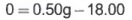Explanation: Always work inside parentheses first. Only use the distributive property when you cannot simplify what is inside the parentheses.

Study Tip: 1. You may want to review the order of operations on page 5. 2. Write these important definitions and rules on note cards and use them to do your homework.

Example 7. Use the distributive property.
3(6x - 5) = Can't combine terms inside the parentheses because they are unlike terms.
3(6x) - 3(5) = Using the distributive property, multiply 6x and -5 by 3.
8x - 15 Cannot combine these unlike terms.

Example 8. Use the distributive property. -5(4x + 2) = Can't combine terms inside the parentheses because they are unlike terms.
(-5)(4x) + (-5)(2) = Using the distributive property, multiply 4x and 2 by -5. -20x -10 Cannot combine these unlike terms.

### Simplifying Algebraic Expressions:

Example 10. Simplify the expression.

4-(2x-3)=
In the same way that -x means -1*x, -(2x-3) means -1(2x-3)
4-2x+3 =
Using the distributive property; multiply 2x and -3 by -1
-2x+72
Combine like terms.

Example 11. Simplify the expression.

11x-14x-3(4x-2)=
Using the distributive property, multiply 4x and -2 by -3.
11x-14-12x+6=
Combine like terms, 11x and -12x, and -14 and 6.
-1x-8=
-1x means the same as -x
-x-8
Cannot combine these unlike terms.

### Summary:

Simplifying Algebraic Expressions
We are now ready to do the real work of algebra. Key definitions include:
1. The distributive property.
a(b + c)= a * b + a * c
The factor "a" multiplies both "b" and "c" inside the parentheses.
2. Terms are separated by addition or subtraction signs.
3. Like terms have the same variable and exponent.
4. Coefficient is the number multiplying the variable.
5. Factors are items being multiplied.

An important last question:
Vocabulary: What is an algebraic expression? An algebraic expression consists of terms, some of which contain variables.

## SOLVING EQUATIONS

### Objectives:

In "Introduction to Variables", we solved equations by guessing. In this section, you will learn how to solve equations using algebra. Algebra is easier and more precise than guessing.

Example 1. You need to rent a moving van. Class Movers charges a basic rate of \$24.95 plus 32 cents per mile.

a. Calculate the cost of renting a van if you drive the following miles.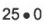The cost equation is c = 0.32m + 24.95.

b. Use the equation to calculate how many miles you drove if the cost is \$42.87.

Logical Solution. The cost \$42.87 contains the basic rate \$24.95. Subtracting \$24.95 from \$42.87 yields \$17.92. This is how much of the cost is attributed to the number of miles driven. Since it costs 32 cents per mile, dividing 17.92 by 0.32 determines the number of miles driven or 56 miles. This same logic is algebra.

Algebraic Solution: Find m when c = 42.87.

42.87 = 0.32m +24.95
Substitute 42.87 for c.
42.87 - 24.95 = 0.32m
Subtract 24.95 from 42.87 becasue the cost, 42.87 contains the basic rate 24.95
17.92 = 0.32m
Combine like terms, 42.87 and 24.95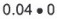The miles driven cost 17.92. Since it costs 32 cents per mile, divide 17.92 by 0.32
56= m

You can drive 56 miles for \$42.87.

c. Check your answer. Substitute m = 56 into the equation c = 0.32m + 24.95; the cost should be \$42.87.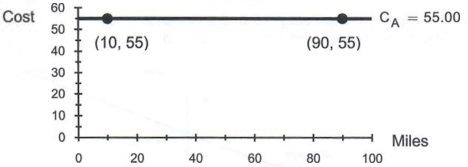Study Tip: You should answer the problem with a sentence, like example 1 above, (You can drive 56 miles for \$42.87.) that refers to both variables in the problem.

Rule: How to solve an equation:
1. Simplify each side of the equation by using the distributive property and combining like terms.
2. The next objective is to write the equation in the form:
Variable term = constant
This is done by using the addition or subtraction properties of equations.
3. Divide both sides by the coefficient (the number multiplying the variable) of the variable.

Explanation: a variable term contains a letter that can represent different values. A constant is a number that never changes value.

Example 2. Solve.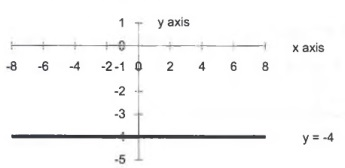Subtract 24.95 from both sides of the equation.
Combine like terms, 42.87 and - 24.95, 24.95 and - 24.95.
This is our first objective in solving the problem.
Divide both sides by the coefficient of m, 0.32
Perform the division.

Example 3. Solve.

7x - 4 = 5(2x + 9) + 3x
Use the distributive property; multiply 2x and 9 by 5.
7x - 4 = 10x + 45 + 3x
Combine like terms; add 10x and 3x
Need a variable term equal to a constant term. Since there is a constant term on both sides of the equation, add 4 to both sides of the equation.
7x-4 = 13x + 45
7x - 4 + 4 = 13x + 45 + 4
Combine like terms, -4 and 4, 45 and 4
7x = 13x + 49
Need a variable term equal to a constant term. Since there is a variable term on both sides of the equation,subtract 13x from both sides of the equation.
7x - 13x = 13x - 13x + 49
Combine like terms, 7x and -13x, 13x and -13x.
-6x=49
Now there is a variable term equal to a constant term,
so divide both sides by -6, the coefficient of x.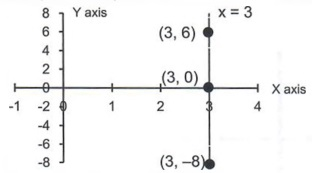Divide.
x=-8.167
Answer is rounded to 3 decimal places.

Example 4. Solve.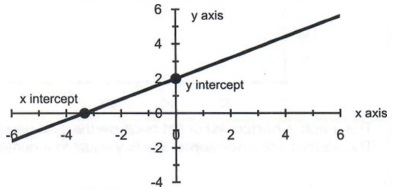Use the distributive property; multiply
6x and -7 by -2.
Combine like terms, 4 and 14.
Need a variable term equal to a constant term. Since there is a variable term on both sides of the equation, add 12x to both sides of the equation.
Combine like terms.
18 can never equal 10, so the conclusion is, the problem has no solution.

Explanation: Look at -12x4-18 = -12x4-10 Notice that-12x is on both sides of the equation, but one side has 18 and the other 10. Do you see why you can't find a solution?

Example 5. Solve.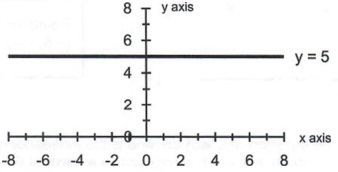Use the distributive property. Combine like terms. Need variable term equal to a constant term. Since there is a variable term on both sides of the equation, subtract 31 x from both sides.
Combine like terms.
-10 is always equal to -10, so the conclusion Is that every number is a solution.

Explanation: Look at -31x -10 = -31x- 10 Notice that the same algebraic expressions are on both sides of the equal sign. Do you see why any number will make the equation true?

Vocabulary: A conditional equation has a finite number of solutions. In this section, a conditional equation will have one solution. Examples 1,2, and 3 are conditional equations.
b. When an equation doesn't have a solution, it is called a contradiction.
Example 4 is a contradiction because when all of the variables are eliminated there is a false arithmetic statement.
c. When all numbers are solutions to an equation, then it is called an identity.
Example 5 is an identity because when all of the variables are eliminated there is a true arithmetic statement.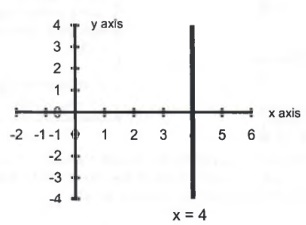Explanation: Solving equations rests on the principle of equality. The principle of equality states: in order to preserve the equality, whatever you do to one side of the equation you must do to the other. In this example, 5 was added to both sides; 2x was subtracted from both sides, and both sides were divided by 4.

### Summary:

Algebra and arithmetic are different. Arithmetic involves operations with numbers. Algebra has variables that can represent many different numbers. If you think back to the section, Introduction to Variables, in the last row of the tables we created, we used variables to represent all of the arithmetic in the previous rows. Algebra is the generalization of repeated arithmetic operations. The equations from Introduction to Variables contained two variables. If you know a value for one of the variables, then you can use the procedures in this section to find the value of the other. Solving equations is a basic function of algebra.

How to solve equations:
1. Simplify both sides of the equation by using the distributive property,
a(b + c) = ab + ac, and combining like terms.
2. The first goal is to write the equation in the form:
Variable term = constant
This is done by using the addition and subtraction principles.
3. Divide both sides of the equation by the coefficient, the number multiplying the variable.
4. The solution to an equation for this type has three possible outcomes:
a. One solution, a conditional equation.
c. All numbers are solutions, or the problem has an infinite number of solutions, an identity.

Study Tip: You should write the steps on a note card along with an example.

## APPLICATIONS OF LINEAR EQUATIONS

### Objective:

This section is a review of the course to date. You will create tables to find equations and then solve them using algebra.

Study Tip: If you have trouble with this section, review Section "Introduction to Variables" and Section "Solving Equations".

Example 1. A student buys a new car in 2010 for \$36,000, and the car depreciates \$3,100 per year.
a. Write an equation that relates the value of the car to the car's age.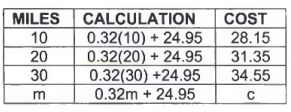The equation is v = 36,000 - 3,1 OOt. t is the number of years since 2010.

b. When will the car be worth \$20,000?
Find y when v = 20,000.
20,000 = 36,000 - 3,100t
Substituted 20,000 for v.
-16,000 = - 3,100t
Subtracted 36,000 from both sides.
5.161 = t
Divided both sides by-3,100.

The car will be worth approximately \$20,000 in 5 years, namely 2015 (2010 + 5).

c. When will the car be worthless?

A car is worthless when its value is zero. Find y when v = 0.
0 = 36,000 - 3,100t
Substituted 0 for v.
3,1 OOt = 36,000
Added 3,1 OOt to both sides,
t = 11.61
Divided both sides by 3,100.

The car will be worthless in about 12 years, namely 2022 (2010 + 12).

Study Tip: In the last problem, we wrote down fewer steps. You should take a couple of minutes to work out the problem in detail. You must know how each step was done. Use your homework notebook. Allow plenty of space so you don't get confused.

Example 2. Class Truck rental company charges a basic rate of \$34.99 plus \$0.20 a mile after the first 15 miles.

a. Write an equation for the cost of renting from Class.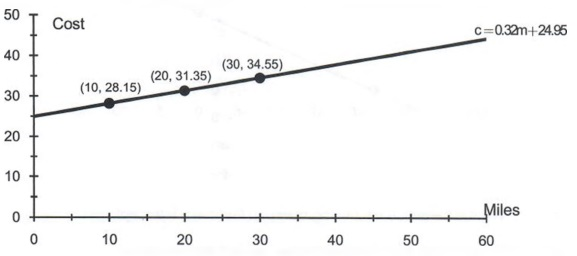Explanation: They charge 20 cents after 15 miles, so if you drive for 25 miles you are only charged 20 cents for 10 miles, 25 - 15. Since you subtracted 25-15 before you multiplied by 0.20, you need parentheses.

The cost equation is c = 0.20(m - 15) + 34.99.

b. Simplify the equation.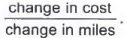Used the distributive property; multiplied m and -15 by
0.20, 0.20m and 0.20(-15) = -3
Combined like terms, -3 and 34.99.

c. How many miles can you go if the charge is \$85? Find m when C = 85.
85 = 0.20m + 31.99
Substituted 85 for C.
53.01 = 0.20m
Subtracted 31.99 from both sides.
265.05 = m
Divided both sides by 0.20.

Rounding to the nearest mile, you can drive approximately 265 miles for \$85.

### Summary:

This section reviews most of the material presented thus far in the course and, therefore, is extremely important. You should be able to:

1. Read the problem and create a table in order to find the equation.

2. Solve algebraic equations to obtain the desired solution. We have advanced to a level of difficulty where "guessing" is a time consuming method for determining algebraic solutions; use algebra.

Study Tips: 1. If any of the above steps is not clear to you, ask questions in the next class session, see your instructor during office hours, go to the tutoring center, or use online tutors.
2. Do not proceed until you have mastered this section.

## LITERAL EQUATIONS

### Objectives:

In this section, you will learn how to solve equations that have two variables. The algebra is the same as in the previous sections. The difference is that the solution will be an equation not a number.

Vocabulary: A literal equation is an equation that involves more than one variable.

Example 1. A cell phone company charges a basic rate of \$1.25 plus \$0.15 per minute after the first 10 minutes.

a. Complete the table to find the cost of making phone calls that last longer than ten minutes.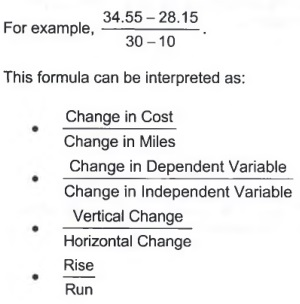Explanation: Parentheses are needed because the charge is not 15 cents per minute until after 10 minutes.

b. What is the equation that relates cost and minutes? Simplify the equation.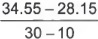Distributive property: multiplied m and -10 by 0.15 Combined like terms, -1.50 + 1.25

c. If the call costs \$2.90, how long were you on the phone? Find m when c = 2.90.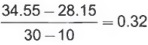Sub 2.90 for c.
2.15 = 0.15m
21 = m.
Divided both sides by 0.15

d. Solve for m in the equation from Part b.

(This is the only new information in this section.)

Why would you want to do this? Imagine a situation where you know the cost of ten different calls. Instead of solving for C ten separate times, you can solve for C once and then use arithmetic to find the ten different values of m.

c = 0.15m - 0.25
c + 0.25 = 0.15m